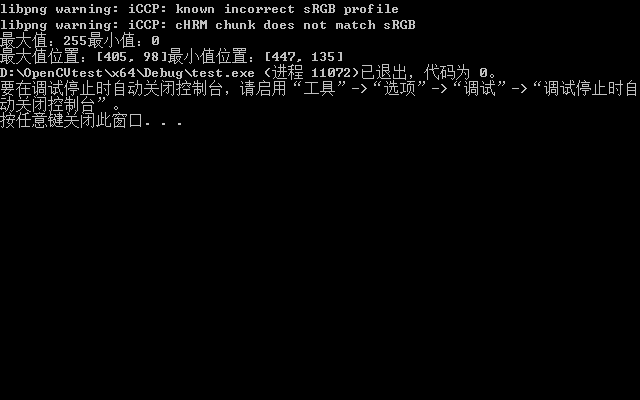• mysql的最大值函数max取不到最大值，这时只需要加个0就行，如下： SELECT MAX(company_code+0) from uw_company ORDER BY company_code desc
mysql的最大值函数max取不到最大值，这时只需要加个0就行，如下：

SELECT MAX(company_code+0) from uw_company ORDER BY company_code desc

展开全文• #Python3 实例--Python 获取最大值函数： print("Python3 实例--Python 获取最大值函数：") def max_num(x, y): print("{}是最大值".format(max(x, y))) max_num(int(input()), int(input())) #运行结果如下： ...
#代码如下：
#Python3 实例--Python 获取最大值函数：
print("Python3 实例--Python 获取最大值函数：")

def max_num(x, y):
print("{}是最大值".format(max(x, y)))

max_num(int(input()), int(input()))

#运行结果如下：
Python3 实例–Python 获取最大值函数：
6
9
9是最大值


展开全文python
• 初学python：最大值函数max的使用
#比较两个或两个以上数字的大小
print("1和2之间的最大值为：",max(1.5,2.5))
#比较两个或多个字符
print("a和b之间的最大值为:",max('a','b','c'))
#也可以用于求列表中的最大值
print("列表[3,4]的最大值为：{}".format(max([3,4])))
#用于求元组中的最大值
print("元组（5,6）的最大值为",max((5,6)))

运行结果：


展开全文• OpenCV 找出图像中最小值最大值函数minMaxLoc的使用 功能：从一个矩阵中找出全局的最大值和最小值。 函数cv::minMaxLoc找出最大和最小元素的值和他们的位置。极值是遍历整个矩阵找到，或者当掩码不是一个空矩阵时...


功能：从一个矩阵中找出全局的最大值和最小值。

函数cv::minMaxLoc找出最大和最小元素的值和他们的位置。极值是遍历整个矩阵找到，或者当掩码不是一个空矩阵时，是通过遍历指定矩阵区域找到。

函数不适用于多通道矩阵，如果需要遍历所有的通道来找到最大和最小值，首先使用函数Mat::reshape转换为单通道矩阵。或者你可以使用函数 extractImageCOI ，mixChannels , or split 来提取特定通道。

函数原型1：

CV_EXPORTS_W void minMaxLoc(InputArray src, CV_OUT double* minVal,
CV_OUT double* maxVal = 0, CV_OUT Point* minLoc = 0,
CV_OUT Point* maxLoc = 0, InputArray mask = noArray());

参数说明：

参数1 src 输入单通道矩阵.

参数1 minVal 返回最小值的指针; 如果不需要输入NULL.

参数 maxVal 返回最大值的指针; 如果不需要输入NULL.

参数 minLoc 返回最小值位置的指针 (二维情况下); 如果不需要输入NULL.

参数 maxLoc 返回最大值位置的指针 (二维情况下); 如果不需要输入NULL.

参考 max, min, compare, inRange, extractImageCOI, mixChannels, split, Mat::reshape

函数原型2：

CV_EXPORTS void minMaxIdx(InputArray src, double* minVal, double* maxVal = 0,
int* minIdx = 0, int* maxIdx = 0, InputArray mask = noArray());

参数说明：

参数1 src 输入单通道矩阵.

参数1 minVal 返回最小值的指针; 如果不需要输入NULL.

参数 maxVal 返回最大值的指针; 如果不需要输入NULL.

参数 minIdx 返回最小值位置的指针 ((n维情况下); 如果不需要输入NULL. 否则,它必须指向src.dims元素的一个矩阵, 每个维度里最小元素的坐标按顺序存储.

参数 maxIdx 返回最大值位置的指针 ((n维情况下); 如果不需要输入NULL.

注意：

在稀疏矩阵中,最小值是只能在非零元素中找到。

当minIdx 不是NULL的时候，他必须有至少两个元素（同maxIdx），即使src是一个单行或者单列矩阵。在OpenCV （跟随MATLAB）中每个矩阵至少要有两个维度。单列矩阵是Mx1 矩阵（因此 minIdx/maxIdx将是(i1,0)/(i2,0)），单行矩阵是 1xN矩阵（因此minIdx/maxIdx 将是(0,j1)/(0,j2)）。

函数原型3：

CV_EXPORTS void minMaxLoc(const SparseMat& a, double* minVal,
double* maxVal, int* minIdx = 0, int* maxIdx = 0);

 

参数说明：

参数1 a 输入单通道矩阵.

参数2 minVal 返回最小值的指针; 如果不需要输入NULL.

参数3 maxVal 返回最大值的指针; 如果不需要输入NULL.

参数4 minIdx 返回最小值位置的指针 ((n维情况下); 如果不需要输入NULL. 否则,它必须指向src.dims元素的一个矩阵, 每个维度里最小元素的坐标按顺序存储.

参数5 maxIdx 返回最大值位置的指针 ((n维情况下); 如果不需要输入NULL.

代码示例：

#include <iostream>
#include <opencv2/opencv.hpp>
​
int main()
{
// std::cout << "Hello World!\n";
cv::Mat image_re = image.reshape(1);
double minValue, maxValue;    // 最大值，最小值
cv::Point  minIdx, maxIdx;    // 最小值坐标，最大值坐标
cv::minMaxLoc(image_re, &minValue, &maxValue, &minIdx, &maxIdx);
std::cout << "最大值：" << maxValue <<"最小值："<<minValue<<std::endl;
std::cout << "最大值位置：" << maxIdx << "最小值位置：" << minIdx;

cv::waitKey(0);
​
}

运行结果如下Mat类的函数reshape

功能：不用拷贝数据转换一个2维矩阵的形状或通道的个数

函数为这些元素制造了一个新的矩阵头，新的矩阵可能会有不同的大小或不同的通道数，如果满足下面条件任何组合都有可能：

没有额外的元素被包含进去新矩阵，也没有元素被排除。所以结果的行数、列数、通道数必须在转换后保持不变。

没有数据拷贝，也就是说这是个 O(1)操作。因此，如果你改变了行的个数，或者操作以其他方式改变了元素行的指数，矩阵必须是连续的，参考：Mat::isContinuous

例如：假如有一些3维的点的集合存储在一个STL vector中，你想用一个3xN矩阵表示这些点，按下面这样做：

std::vector<Point3f> vec;
...
Mat pointMat = Mat(vec). // convrt vector to Mat, O(1) operation
reshape(1). // make Nx3 1-channel matrix out of Nx1 3-channel.
// Also, an O(1) operation
t(); // finally, transpose the Nx3 matrix.
// This involves copying all the elements

函数原型1：

Mat reshape(int cn, int rows=0) const;

参数说明：

参数1 cn 新通道数.如果参数是0，通道数保持不变.

参数2 rows 新通道行数. 如果参数是0，行数保持不变.

函数原型2：

Mat reshape(int cn, int newndims, const int* newsz) const;

函数原型3：

Mat reshape(int cn, const std::vector<int>& newshape) const;


展开全文opencv
• 6-4 最大值函数模板(*) (10分) 请设计求两个对象最大值的函数模板。 #include <iostream> using namespace std; /* 你提交的代码将被嵌在这里 */ int main() { int a, b, c; cin >> a >> b...
• int Max(int a, int b, int c)//求最大值函数的实现 { int max = 0; if (a > max) max = a; if (b > max) max = b; if (c > max) max = c; }; int main(int* argc, char* argv[]) { int num1, ...c语言
• 最大值函数:从键盘输入两个double类型数据，a,b，通过定义并调用函数max_ab实现求a与b的最大值输出。c c++ 编程语言
• Hibernate（分组查询等续）  ...Max：求最大值函数 Sum：求和函数 Avg：求平均数函数  Count：统计函数 Session session = HibernateSessionFactory.getSession(); Transaction tx = ses
• 语法格式：db.getCollection('集合名称(表名)').aggregate([{$group:{_id: '$分组求值目标域(字段)',结果域名称:{$max:"$文档中域名称"}}}]);...最大值db.getCollection('logo').aggregate([{\$group:{...mongodb
• #include<stdio.h> #include<stdlib.h> int GetMax(int m, int n) { if (m > n) { return m; } else { return n;...printf("两个数值之间最大是：%d\n",resul..c语言
• 题目解决代码及点评#include ...编写一函数求三个整数的最大值。 */ void f62(int a ,int b,int c) { int temp=a; if (temp) { temp=b; } if (temp) { temp=c; } printf("最大数是%d\n",temp); } void
• #include int maxElement(array[] ,int n) {  int i=0,n=0,t=array;//将下标为0的赋值给t  for(i=0;...//统计比较次数 假设a最大则不比较，则下标最大者为a,假设a最大则循环要执
• ## (C语言)用函数实现求三个数最大值

万次阅读 多人点赞 2018-12-08 23:07:34
题目描述：从键盘输入三个数，之后调用函数实现求三个数的最大值 ... //求最大值函数的声明 int x,y,z; int max_value;//用来存最大值 scanf("%d%d%d",&amp;x,&amp;y,&am...
•最小值
• python接收多个数字，返回最大值自定义函数， 多谢@Gavin_Hall 为我解答我的疑问 max_num = 0 def my_max(x,y): #简单比较两个数大小 global max_num #申请全局变量max_num if x > y: max_num = x else: ...python
• 自定义查找最大值函数findMax()，功能：查找给定区域内的最大值（按字典排序，数字小于字母，大写字母小于小写字母）代码：Function findMax(ByVal rng As Range) '求最大值 Dim max As Variant max = rng.Cells(1)....
•  EXCEL中MAX和MIN函数最大值和最小值，是函数使用过程中经常用于处理数值的两个函数，通过这两个函数，可以轻松处理一串数值，确定其最值并且MAX和MIN函数可作为数组函数使用。  源数据我们依然使用以前的数据...excel MAX 数组
• 具体如图： 可以看出：Maximize和MaxValue求得是精确解，并且Maximize给出最大值时变量的取值，而FindMaxValue给的数值解（非精确解）。
• 假设要在程序中实现求最大值，但是由于求最大值的个数和参数都不确定，因此要针对不同的情况设计不同的方法，这里就用到了函数重载。函数重载函数名相同但是参数的类型和个数不能相同。下面的例子分别是求两个数的...java
• ## 函数重载求最大值

千次阅读 2016-11-19 21:22:11
利用重载函数实现：分别求出2个double型、3个double型或4个double型数中最大值和最小值，并在main函数中调用之。小问题：返回两个值，考虑实参引用& ；子函数在return之后就不再运行；用嵌套三目的方法，选一个参数...
• 任意从键盘输入10个整数，用函数编程实现计算其中的最大值。 要求用数组作为函数参数编程实现计算最大值函数FindMax()，即按照如下函数原型编写该函数：int FindMax(int num[], int n);要求：1）在主函数中打印...
• 函数的定义： 一个凸集内的任意两个点x1与x2，任意t∈[0,1]⇒f(tx1+(1−t)x2)≤tf(x1)+(1−t)f(x2)一个凸集内的任意两个点x1与x2，任意t∈[0,1]⇒f(tx1+(1−t)x2)≤tf(x1)+(1−t)f(x2)一个凸集内的任意两个点 x_1...
• #include #include using namespace std; int main() { int n[]={1,4,22,3,8,5}; int len=sizeof(n)/sizeof(int); //数组长度 cout*max_element(n,n+len); //找最大值 最小值 cout*min_element(n,n
• a =  1 2  5 6  5 5  4 5 >> max(a) ans =  5 6 >> max(a(2:3,:)) ans =  5 6 >> max(a(3:4,:)) ans =
• sql函数平均值 总数 最小值 最大值 总和 标准差
• 输入格式:111 222 111 222中间敲一个空格。这个空格你敲了吗？见图片12580 空格 12581，自己调试的时候两个数之间敲一个空格~
• python中 .max() 函数在对 array 和 tensor 作用不同。...1. array数组使用max的效果是返回最大值 eg： zheshi = np.array([[1,2,3], [4,5,6]]) 返回： array([[1, 2, 3], [4, 5, 6]]) zheshi.max(0)...数组 tensor
• 声明一个求两个数最大值函数 if(a>b){ printf("最大值是 %d",a); } else{ printf("最大值是 %d",b); } */ #include <stdio.h> max(int a,int b){ //三木运算来求出最大值 int z...
• 编写函数求一个数组中数组元素的最大值，要求必须用递归方法解决。 输入格式: 数组中的数字均为整型数，输入的第一个数为数组长度，后续为数组的所有元素。 输出格式： 该数组的最大值 输入样例： 5 92 18 77......